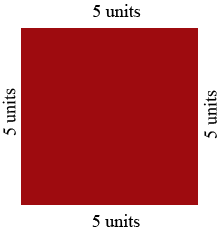SEARCH HOMEMath Central Quandaries & QueriesQuestion from Bryan, a student: Find an expression that represents the area of a square with side length 4x-3Hi Bryan,

Suppose you had a square and each side measures 5 units.The area of thus square is then $5 \times 5 = 25$ square units.

Now perform the same operation when each side is of length $4x-3$ units. For my square I expanded $5 \times 5$ to get $25.$ What do you get when you expand the area expression for a square with sides of length $4x-3$ units?

PennyMath Central is supported by the University of Regina and the Imperial Oil Foundation.